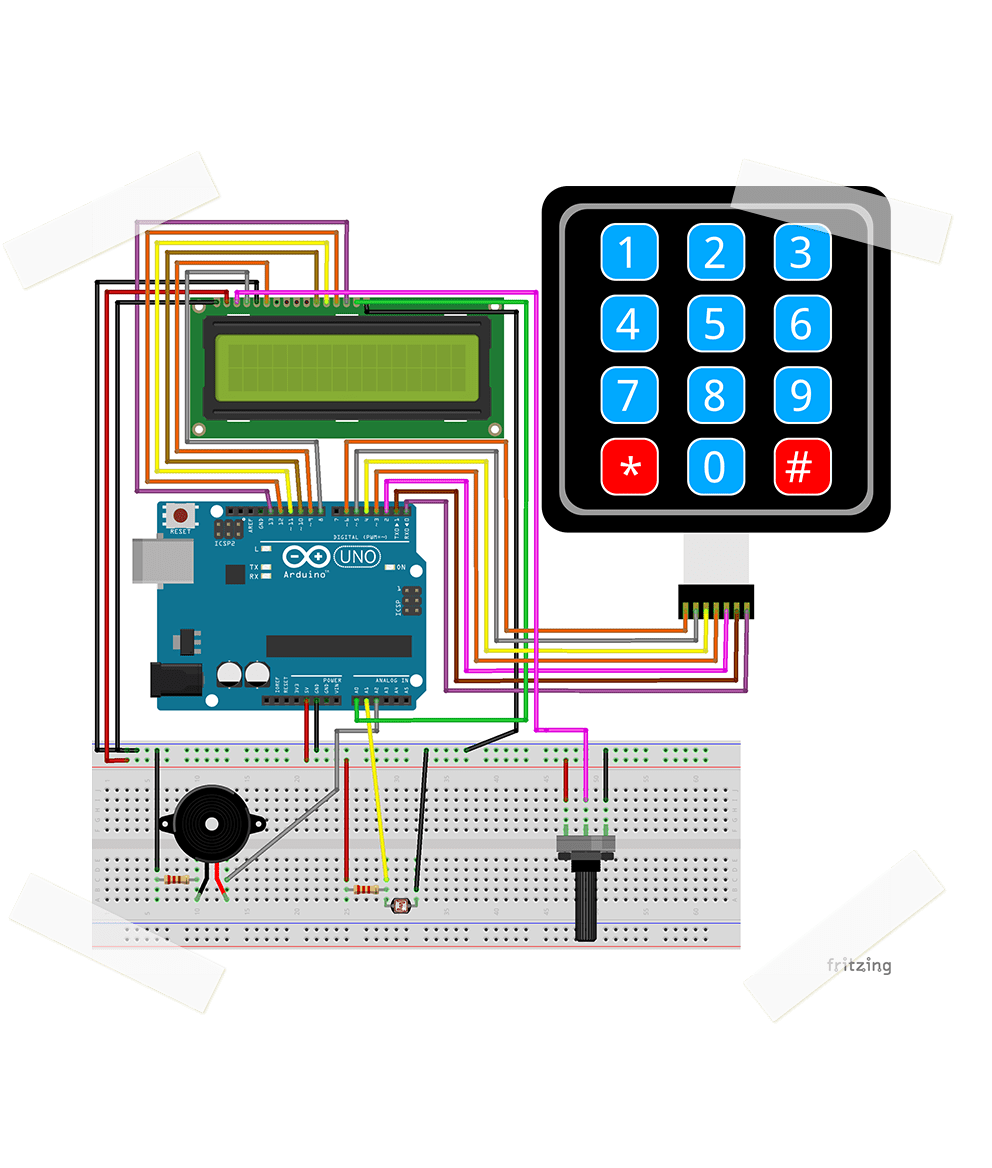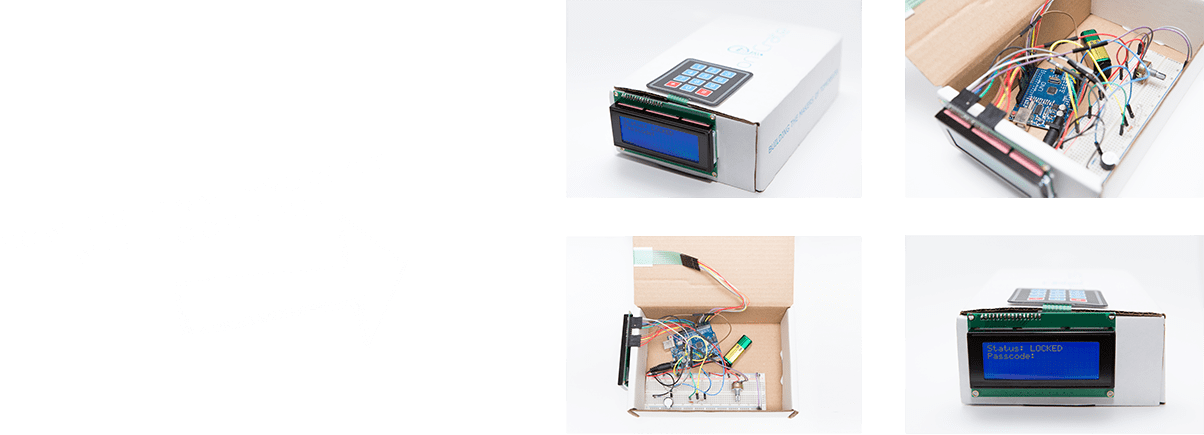# Lock Box# EXERCISE SOLUTIONS:

Question 1

This solution will utilize the millis() command. This command returns the time in milliseconds since the Uno R3 was turned on. We can use this by saving the time in a variable, and comparing the variable to the current time to determine elapsed time.

First, we will add two variables. One will hold the time at which the device was last unlocked. The other will be a constant for how many milliseconds until the device is locked again. To turn seconds into milliseconds, multiply by 1000. The code below sets the timeout to 60 seconds, which is 60,000 milliseconds.

long unlockTime;
const long lockTimeout = 60000;

Next, we add the line unlockTime = millis(); in the following if statement:

if(String(inputList) == passcode) {
unlockTime = millis();
unlocked = true;

Lastly, we add the following if statement to the loop() function:

if(unlocked)
{
if(millis() > lockTimeout + unlockTime)
{
unlocked = false;
lightLCD = false;
setScreen();
}
}

Question 2

To do exercise 2, we can add the following lines of code to the inputKey if statement:

if(inputKey) {
digitalWrite(BUZZER, HIGH);
delay(50);
digitalWrite(BUZZER, LOW);

Question 3

To be able to change the password, we will need a new char array and a new int:

char passInputList; // Holds the new passcode.
int passInputIndex = 0; // How many digits have been entered.

We need to modify the inputKey if statement, and add a passInputIndex if statement. The entire new void loop is shown in 'Q3 Void Loop.ino'. Download it in the 'I Give Up' file.

Bonus Question

We need to switch the LCD backlight pin with a pin that has PWM capability. We will use pin 6 in this example. In the beginning of the code, swap the two out.

// Pins for other sensors.
#define BUZZER A2
#define LDR A1
#define BACKLIGHT 6

// The pin assignment for the matrix keypad.
byte rowPins{ROWS] = {A0,5,4,3};
byte colPins[COLS] = {2,1,0};

Then, in the hardware, switch the wires connected to pin 6 and pin A0. In the software, replace digitalWrite(BACKLIGHT) with analogWrite():

if(lightLCD) {
analogWrite(BACKLIGHT, 100);
}
else {
analogWrite(BACKLIGHT, 0);
}
}